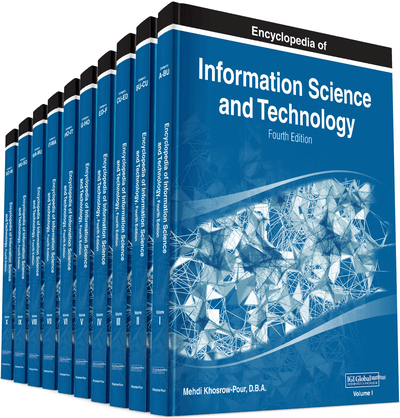# Evolutionary Algorithms for Global Decision Tree Induction

DOI: 10.4018/978-1-5225-2255-3.ch185
OnDemand:
(Individual Chapters)
Available
\$37.50
No Current Special Offers

## Abstract

Decision trees represent one of the main predictive techniques in knowledge discovery. This chapter describes evolutionary induced trees, which are emerging alternatives to the greedy top-down solutions. Most typical tree-based system searches only for locally optimal decisions at each node and do not guarantee the optimal solution. Application of evolutionary algorithms to the problem of decision tree induction allows searching for the structure of the tree, tests in internal nodes and regression functions in the leaves (for model trees) at the same time. As a result, such globally induced decision tree is able to avoid local optima and usually leads to better prediction than the greedy counterparts.
Chapter Preview
Top

## Background

We may find different variants of decision trees in the literature (Loh, 2014). They can be grouped according to the type of problem they are applied to, the way they are induced, or the type of structure. Tree predictors can be used to classify existing data (classification trees) or to approximate real-valued functions (regression trees) (see Figure 1). In each leaf, classification tree assigns a class label (usually the majority class of all instances that reach that particular leaf), while the regression tree holds a constant value (usually an average value for the target attribute). A model tree can be seen as an extension of the typical regression tree (Quinlan, 1992). The constant value in each leaf of the regression tree is replaced in the model tree by a linear (or nonlinear) regression function. To predict the target value, the new tested instance is followed down the tree from a root node to a leaf using its attribute values to make routing decisions at each internal node. Next, the predicted value for the new instance is evaluated based on a regression model in the leaf.

Examples of predicted values of classification, regression, and model trees are given in Figure 1.

The gray level color of each region represents a different class label (for a classification tree), and the height corresponds to the value of the prediction function (regression and model trees).

## Key Terms in this Chapter

Decision Tree Pruning: An approach that reduces the size of decision tree by removing parts of the tree that provide little power to classify/predict the data. This technique improves the generalization power of the decision tree and reduces the over-fitting.

Multivariate Decision Tree: A decision tree with tests involving several attributes. The most common form of such tests is an oblique split, which is based on a linear combination of features (hyper-plane).

Top-Down Induction: A recursive method of decision tree generation. It starts with the entire input dataset in the root node where a locally optimal test for data splitting is searched and branches corresponding to the test outcomes are created. The test searches and data splitting are repeated in the created nodes unless the stopping condition is met.

Model Tree: An extension of regression trees were the constant value in the terminal node is replaced by the regression plane.

Global Induction: A method of decision tree generation, where both the tree structure and all tests are searched at the same time; usually based on evolutionary approach in contrast to top-down induction.

Mixed Decision Tree: A decision tree with heterogeneous representation. The tests in internal nodes may be univariate or multivariate whereas the leaves may hold a constant value or a regression model.

Univariate Decision Tree: A decision tree which partitions the feature space with axis-parallel hyper-planes. Each split at non-terminal node involves single feature.

Decision Tree: A decision tree is a graph that uses a branching method to illustrate every possible outcome of a decision. Each branch of the decision tree represents a possible decision or occurrence. The tree structure shows how one choice leads to the next, and the use of branches indicates that each option is mutually exclusive.

## Complete Chapter List

Search this Book:
Reset